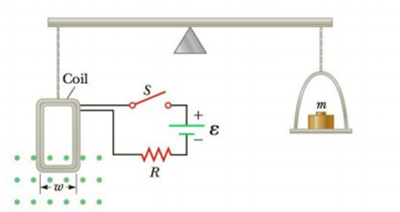Chapter 19, Problem 64AP

Chapter
Section
Textbook Problem

Figure P19.64 is a setup that can be used to measure magnetic fields. A rectangular coil of wire contains N turns and has a width w. The coil is attached to one arm of a balance and is suspended between the poles of a magnet. The field is uniform and perpendicular to the plane of the coil. The system is first balanced when the current in the coil is zero. When the switch is closed and the coil carries a current I, a mass m must be added to the right side to balance the system. (a) Find an expression for the magnitude of the magnetic field and determine its direction. (b) Why is the result independent of the vertical dimension of the coil? (c) Suppose the coil has 50 turns and width of 5.0 cm. When the switch is closed, the coil carries a current of 0.30 A, and a mass of 20.0 g must be added to the right side to balance the system. What is the magnitude of the magnetic field?Figure P19.64

(a)

To determine
The expression of the magnitude of the magnetic field and to find the direction of the magnetic field.

Explanation

Given Info: The number of turns in the rectangular coil is N . The width of the loop is w . The coil is suspended between the poles of a magnet. The field is uniform and perpendicular to the plane of the coil. The system is balanced in the first when the current in the coil is zero.

Explanation:

When the switch S is closed a current of NI flows throught the bottom arm of the coil toward the right. The magnetic force has to be downward in order to be in balance condition.

Since the magnetic field is perpendicular to the plane of the coil the magnitude of the downward force will be,

Fm=BNIw

• B is the magnetic field
• w is the the width of the coil
• NI is the total current flowing in the loop

When the system is in balance condition the downward manetic force must be equal to the gravitational force due to the mass present in the other side of the balance

(b)

To determine
The reason why the magnetic field is independent of the vertical dimension of the coil.

(c)

To determine
The magnitude of the magnetic field.

Still sussing out bartleby?

Check out a sample textbook solution.

See a sample solution

The Solution to Your Study Problems

Bartleby provides explanations to thousands of textbook problems written by our experts, many with advanced degrees!

Get Started